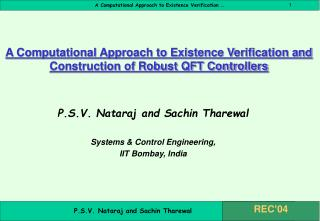Download PresentationA Computational Approach to Existence Verification and Construction of Robust QFT Controllers

# A Computational Approach to Existence Verification and Construction of Robust QFT Controllers - PowerPoint PPT PresentationDownload Presentation## A Computational Approach to Existence Verification and Construction of Robust QFT Controllers

- - - - - - - - - - - - - - - - - - - - - - - - - - - E N D - - - - - - - - - - - - - - - - - - - - - - - - - - -
##### Presentation Transcript

1. A Computational Approach to Existence Verification and Construction of Robust QFT Controllers P.S.V. Nataraj and Sachin Tharewal Systems & Control Engineering, IIT Bombay, India

2. Outline • Contribution • Introduction to QFT • Problem definition • Proposed Algorithm • Example • Conclusions

3. Contribution • Existence verification • Constructive approach – • Gauranteed existence verification • Give all solutions, if any solution exist.

4. R(s) Y(s) F(s) K(s) G(s) -1 Introduction 2-DOF Structure for QFT formulation

5. Introduction … QFTObjective : Synthesize K(s) and F(s) for the following specifications: • Robust Stability margin • Tracking performance • Disturbance Attenuation

6. Introduction … QFT Procedure : • Generate the plant template at the given design frequencies . • Generate the bounds in terms of nominal plant, at each design frequency, on the Nichols chart.

7. Introduction … QFT Procedure … • Synthesize a controller K(s) such that • The open loop response satisfies the given performance bounds, • And gives a nominal closed loop stable system. • Synthesize a prefilter F(s) which satisfies the closed loop specifications.

8. Problem Definition • Given an uncertain plant and time domain or frequency domain specification, find out if a controller of specified (transfer function) structure exist.

9. . Feasible Ambiguous . Infeasible Feasibility Test . Feasible magnitude Ambiguous . Infeasible phase

10. Proposed Algorithm Inputs: • Numerical bound set, • The discrete design frequency set, • Inclusion function for magnitude and angle, • The initial search space box z0. Output: Set of Controller parameters, OR The message “No solution Exist”.

11. Proposed Algorithm… BEGIN Algorithm • Check the feasibility of initial search box z0. • Feasible  Complete z0is feasible solution. • Infeasible  No solution exist in z0 • Ambiguous  Further processing required : Initialize stack list Lstack and solution list Lsol.

12. Proposed Algorithm … • Choose the first box from the stack list Lstackas the current box z, and delete its entry form the stack list. • Split z along the maximum width direction into two subboxes.

13. Proposed Algorithm … • Find the feasibility of each new subbox: • Feasible  Add to solution list Lsol. • Infeasible  Discard. • Ambiguous  Further processing required : Add to stack list Lstack.

14. Proposed Algorithm • IF Lstack = {empty} THEN (terminate) • Lsol = {empty}  “No solution exist”, Exit. • Lsol {empty}  “Solution set = ” Lsol, Exit. • Go to step 2 (iterate). End Algorithm

15. Example • Uncertain plant : • Uncertainty : • Robust stability spec: • Tracking spec:

16. QFT Bounds

17. Example … • For first order controller: • Parameter vector • Initial search box • For second order controller: • Parameter vector • Initial search box

18. Example … • For third order controller: • Parameter vector • Initial search box • For fourth order controller: • Parameter vector • Initial search box

19. Results • For the aforementioned structures and the initial search domains, the proposed algorithm terminated with the message: “No feasible solution exists in the given initial search domain”. • This finding is in agreement with the analytically found ‘non-existence’ of Horowitz.

20. Conclusions • An algorithm has been proposed to computationally verify the existence (or non-existence) of a QFT controller solution. • Proposed algorithm has been tested successfully on a QFT benchmark example. • The proposed algorithm is based on interval analysis, and hence provides the most reliable technique for existence verification.

21. Thank you !